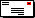### Properties of KTP/KTA Single Crystal

Potassium Titanyl Phosphate, known as KTP( KTiOPO4), and Potassium Titanyl Arsenate, known as KTA(KTiOAsO4), are widely used in frequency doubling of Nd-doped laser systems for Green/Red output; parametric sources(OPG, OPA and OPO) for 600nm-4500nm tunable output; E-O modulators, Optical Switches, Directional Couplers; Optical Waveguides for Integrated NLO and E-O Devices etc.

#### Optical Properties of KTP:

 Transmitting Range: 350nm ~ 4500nm Phase Matching Range: 984nm ~ 3400nm Refractive Indices: @1064nm 1.7377(nx) 1.7453(ny) 1.8297(nz) @532nm 1.7780(nx) 1.7886(ny) 1.8887(nz) Sellmeier Equations:           (l in µm) Nx2 = 3.0065 + 0.03901 / ( l2 - 0.04251) - 0.01327l2 Ny2 = 3.0333 + 0.04154 / ( l2 - 0.04547) - 0.01408l2 Nz2 = 3.3134 + 0.05694 / ( l2 - 0.05658) - 0.01682l2 Therm-Optic Coefficient:(10-5/°C) dnx/dT=1.1 dny/dT=1.3 dnz/dT=1.6 Absorption Coefficient: a<1%/cm @1064nm and 532nm Nonlinear Optical Coefficients and Equation: @1064nm d31=2.54pm/V d32=4.35pm/V d33=16.9pm/V d24=3.64pm/V d15=1.91pm/V deff(II)=(d24-d15)sin2f sin2q - (d15sin2f +d24cos2q )sinq Electro-optic coefficients: Low Frequency(pm/V) High Frequency(pm/V) r13 9.5 8.8 r23 15.7 13.8 r33 36.3 35.0 r51 7.3 6.9 r42 9.3 8.8 Dielectric constant: eeff=13

Physical Properties of KTP:

 Crystal Structure: Orthorhombic, point group mm2 Cell Parameters: a=6.404Å, b=10.616Å, c=12.814Å, z=8.00Å Melting Point: 1172°C Curie Point: 936°C Mohs Hardness: »5 Density: 3.01g/cm3 Color: Colorless Hygroscopic Susceptibility no Specific Heat: 0.1643cal/g°C Thermal Conductivity: 0.13W/cm·K Electrical Conductivity: 3.5x10-8 s/cm (c-axis, 22°C, 1KHz)

#### Optical Properties of KTA:

 Transmitting Range: 350nm ~ 5300nm Phase Matching Range: 1080nm ~ 3790nm Refractive Indices: @1064nm 1.7817(nx) 1.7865(ny) 1.8678(nz) @532nm 1.8263(nx) 1.8329(ny) 1.9308(nz) Absorption Coefficient: a<0.05%/cm @1000nm Nonlinear Optical Coefficients and Equation: @1064nm d31=2.8pm/V d32=4.2pm/V d33=16.2pm/V d24=3.2pm/V d15=2.3pm/V deff(II)=(d24-d15)sin2f sin2q - (d15sin2f +d24cos2q )sinq Electro-optic coefficients: Low Frequency(pm/V) High Frequency(pm/V) r13 11.5 N/A r23 15.4 N/A r33 37.5 N/A Dielectric constant: eeff=42

Physical Properties of KTA:

 Crystal Structure: Orthorhombic, point group mm2 Cell Parameters: a=13.13Å, b=6.57Å, c=10.79Å, z=8.00Å Melting Point: 1130°C Curie Point: 880°C Mohs Hardness: »5 Density: 3.45g/cm3 Color: Colorless Hygroscopic Susceptibility no Specific Heat: 0.687cal/g°C Electrical Conductivity: 0.7~3.4x10-6 s/cm (c-axis, 22°C, 2KHz)

Applications:

Frequency Conversion:

KTP was first introduced as the NLO crystal for Nd doped laser systems with high conversion efficiency. Under certain conditions, the conversion efficiency was reported to 80%, which leaves other NLO crystals far behind.

Recently, with the development of laser diodes, KTP is widely used as SHG devices in diode pumped Nd:YVO4 solid laser systems to output green laser, and also to make the laser system very compact.

KTP for OPA, OPO Applications:

In addition to its wide use as a frequency doubling device in Nd-doped laser systems for Green/Red output, KTP is also one of the most important crystals in parametric sources for tunable output from visible (600nm) to mid-IR (4500nm) due to the popularity of its pumped sources, the fundamental and second harmonic of a Nd:YAG or Nd:YLF lasers.

One of the most useful applications is the non-critical phase-matched (NCPM) KTP OPO/OPA pumped by the tunable lasers to obtain a high conversion efficiency.

KTP OPO results in stable continuous outputs of femto-second pulse of 108 Hz repetition rate and milli-watt average power levels in both signal and idler outputs.

Pumped by Nd-doped lasers, KTP OPO has obtained above 66% conversion efficiency for down-conversion from 1060nm to 2120nm.

Electro-Optical modulators:E-mail this link to a friend